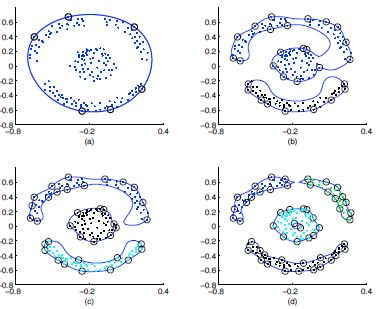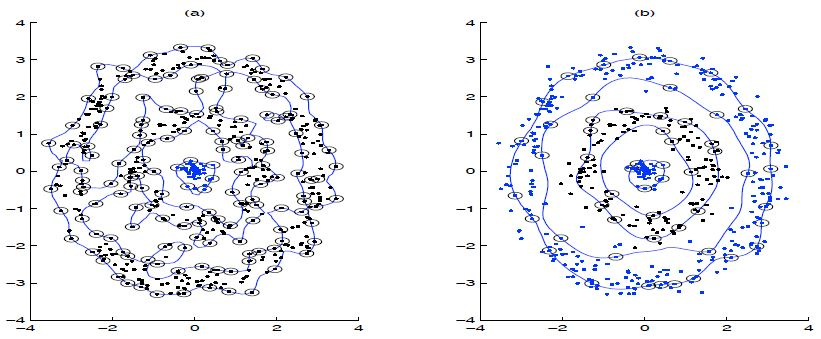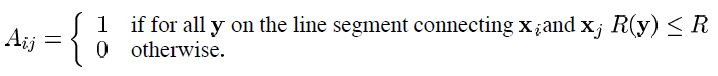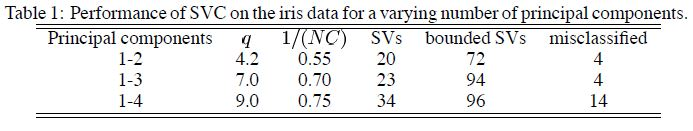# Describing Cluster Boundaries with Support Vectors

$L={R}^{2}-\mathrm{\Sigma }\left({R}^{2}+{\xi }_{j}-{‖\mathrm{\Phi }\left({x}_{j}\right)-a‖}^{2}\right){\beta }_{j}-\mathrm{\Sigma }{\xi }_{j}{\mu }_{j}+C\mathrm{\Sigma }{\xi }_{j},$

$\mathrm{\Sigma }{\beta }_{j}=1,\phantom{\rule{0ex}{0ex}}a=\mathrm{\Sigma }{\beta }_{j}\mathrm{\Phi }\left({x}_{j}\right),\phantom{\rule{0ex}{0ex}}{\beta }_{j}=C-{\mu }_{j}$

KKT条件：

${\xi }_{j}{\mu }_{j}=0,\phantom{\rule{0ex}{0ex}}\left({R}^{2}+{\xi }_{j}-{‖\mathrm{\Phi }\left({x}_{j}\right)-a‖}^{2}\right){\beta }_{j}\right)=0$

• ${\xi }_{i}>0$$\xi _i >0$则样本点在超球外；
• ${\xi }_{i}=0$$\xi _i =0$则样本点在超球面上或超球面内，当${\beta }_{i}\ne 0$$\beta_i \neq0$
• $0<{\beta }_{i}$0<\beta_i则称样本点是一个支持向量（SV）；
• ${\beta }_{i}=C$$\beta_i =C$则称样本点为边界支持向量（bounded SV）

$W={\mathrm{\Sigma }}_{j}\mathrm{\Phi }\left({x}_{j}{\right)}^{2}{\beta }_{j}-{\mathrm{\Sigma }}_{i,j}{\beta }_{i}{\beta }_{j}\mathrm{\Phi }\left({x}_{i}\right)\cdot \mathrm{\Phi }\left({x}_{j}\right)$

$0\le {\beta }_{j}\le C$

$K\left({x}_{i},{x}_{j}\right)={e}^{-q{‖{x}_{i}-{x}_{j}‖}^{2}},$

$q$$q$为宽度参数（width parameter，即$\frac{1}{2{\sigma }^{2}}$$\frac{1}{2\sigma ^2}$），那么拉格朗日对偶函数可写为：

$W={\mathrm{\Sigma }}_{j}K\left({x}_{j},{x}_{j}\right){\beta }_{j}-{\mathrm{\Sigma }}_{i,j}{\beta }_{i}{\beta }_{j}K\left({x}_{i},{x}_{j}\right)。$

${R}^{2}\left(x\right)={‖\mathrm{\Phi }\left({x}_{j}\right)-a‖}^{2}$

${R}^{2}\left(x\right)=K\left(x,x\right)-2{\mathrm{\Sigma }}_{j}{\beta }_{j}K\left({x}_{j},x\right)+{\mathrm{\Sigma }}_{i,j}{\beta }_{i}{\beta }_{j}K\left({x}_{i},{x}_{j}\right)$# Support Vector Clustering (SVC)## Overlapping clusters（不是很懂）

$p\left(x\right)=\frac{1}{N}{\mathrm{\Sigma }}_{i}K\left({x}_{i},x\right)$

Parzen窗概率密度估计值最大的点便是核心的点。

## The iris data# Varying q$q$$q$ and C$C$$C$

©️2019 CSDN 皮肤主题: 大白 设计师: CSDN官方博客